IMO Shortlist 1959 problem 3

Kvaliteta:
Avg: 0,0
Težina:
Avg: 0,0
Let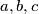$a,b,c$ be real numbers. Consider the quadratic equation in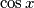$\cos{x}$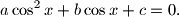Using the numbers$a,b,c$ form a quadratic equation in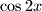$\cos{2x}$ whose roots are the same as those of the original equation. Compare the equation in$\cos{x}$ and$\cos{2x}$ for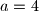$a=4$,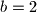$b=2$,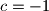$c=-1$.
Izvor: Međunarodna matematička olimpijada, shortlist 1959